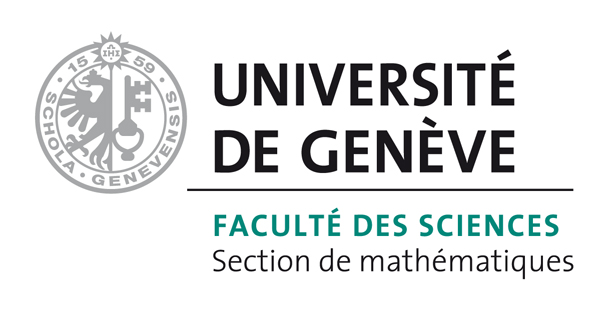# Bart Vandereycken## Greedy rank updates combined with Riemannian descent methods for low-rank optimization

by ,

#### Abstract:

We present a rank-adaptive optimization strategy for finding low-rank solutions of matrix optimization problems involving a quadratic objective function. The algorithm combines a greedy outer iteration that increases the rank and a smooth Riemannian algorithm that further optimizes the cost function on a fixed-rank manifold. While such a strategy is not especially novel, we show that it can be interpreted as a perturbed gradient descent algorithms or as a simple warm-starting strategy of a projected gradient algorithm on the variety of matrices of bounded rank. In addition, our numerical experiments show that the strategy is very efficient for recovering full rank but highly ill-conditioned matrices that have small numerical rank.

#### Reference:

A. Uschmajew, B. Vandereycken, "Greedy rank updates combined with Riemannian descent methods for low-rank optimization", In Sampling Theory and Applications (SampTA), 2015 International Conference on, IEEE, pp. 420-424, 2015.

#### Bibtex Entry:

@inproceedings{Uschmajew_V:2015,
Abstract = {We present a rank-adaptive optimization strategy for finding low-rank solutions of matrix optimization problems involving a quadratic objective function. The algorithm combines a greedy outer iteration that increases the rank and a smooth Riemannian algorithm that further optimizes the cost function on a fixed-rank manifold. While such a strategy is not especially novel, we show that it can be interpreted as a perturbed gradient descent algorithms or as a simple warm-starting strategy of a projected gradient algorithm on the variety of matrices of bounded rank. In addition, our numerical experiments show that the strategy is very efficient for recovering full rank but highly ill-conditioned matrices that have small numerical rank.},
Author = {Uschmajew, A. and Vandereycken, B.},
Booktitle = {Sampling Theory and Applications (SampTA), 2015 International Conference on},
Doi = {10.1109/SAMPTA.2015.7148925},
Pages = {420--424},
Pdf = {http://www.unige.ch/math/vandereycken/papers/published_Uschmajew_V_2015.pdf},
Publisher = {IEEE},
Title = {Greedy rank updates combined with {R}iemannian descent methods for low-rank optimization},
Year = {2015}}